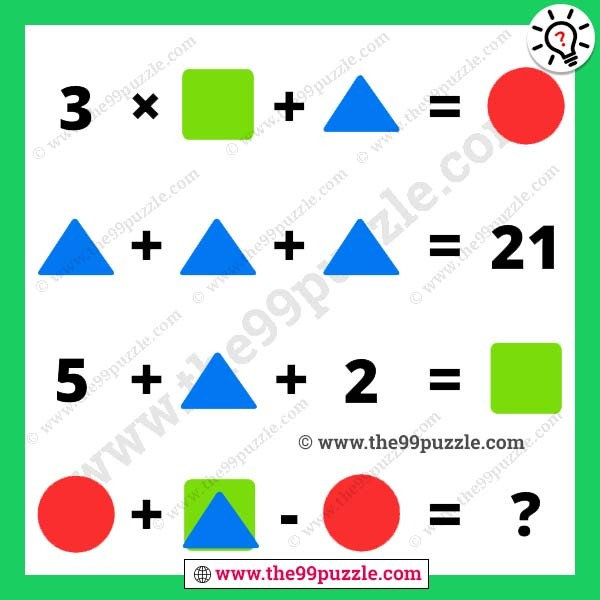# Shape picture math reasoning with answer – Puzz235

Can you quickly answer the shape picture math reasoning? These picture puzzles are very challenging to solve in a minute. 99% of people fail to answer quickly. Only genius and smart people can give the correct answer. Brainteaser picture puzzle cracks your logical mind. Practice puzzles regularly to improve your logic skills. Now, most of the competitive exams ask many brain teasers and logic questions. If you want to gain your puzzles knowledge to practice our all puzzles section. Here, in this picture, you see some shapes with numbers in equation format. You have to find out each value and solve the last picture equation.###### Explanation:

3 × Square + Triangle = Circle

Triangle + Triangle + Triangle = 21

5 + Triangle + 2 = Square

Circle + (Square+Triangle) – Circle = ?

Square = 14

Triangle = 7

Circle = 49

3 × 14 + 7 = 49

7 + 7 + 7 = 21

5 + 7 + 2 = 14

49 + (14+7) – 49 = 21